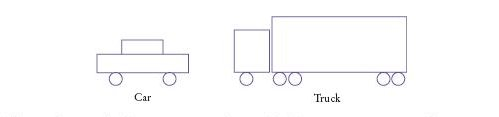Implement an abstract class Vehicle and concrete subclasses Car and Truck. A vehicle has a position on the screen. Write methods draw that draw cars and trucks as follows:Then write a method randomVehicle that randomly generates Vehicle references, with an equal probability for constructing cars and trucks, with random positions. Call it 10 times and draw all of them.

Complete the following files:

### Truck.java

Use the following files:

RandomVehicleViewer.java

```import javax.swing.*;

/**
This program draws cars and trucks in random order.
*/
public class RandomVehicleViewer
{
public static void main(String args[])
{
JFrame frame = new JFrame();
final int FRAME_WIDTH = 600;
final int FRAME_HEIGHT = 600;

frame.setSize(FRAME_WIDTH, FRAME_HEIGHT);
frame.setDefaultCloseOperation(JFrame.EXIT_ON_CLOSE);

RandomVehicleComponent component = new RandomVehicleComponent();
frame.setVisible(true);
}
}
```

Vehicle.java

```import java.awt.Graphics2D;

/**
This class represents a vehicle.
*/
public abstract class Vehicle
{
private int xleft;
private int ytop;

/**
Construct a Vehicle object.
*/
public Vehicle()
{
xleft = 0;
ytop = 0;
}

/**
Draw the specified vehicle.
@param g2 the graphics context
*/
public abstract void draw(Graphics2D g2);

/**
Set the location of the vehicle.
@param x the x coordinate
@param y the y coordinate
*/
public void setLocation(int x, int y)
{
xleft = x;
ytop = y;
}

/**
Returns the x coordinate of the left-top corner of the vehicle
@return the x coordinate
*/
public int getX()
{
return xleft;
}

/**
Returns the y coordinate of the left-top corner of the vehicle
@return the y coordinate
*/
public int getY()
{
return ytop;
}

public abstract int getHeight();
public abstract int getWidth();
}
```